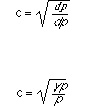# Compression Wave Velocity

Compression Wave Velocity
The general expression for the velocity of a compressional wave in a deformable medium is the first equation below where dp is the change in pressure accompanying the change dp in density. Since the compressions and rarefactions accompanying the propagation of sound through a gas are adiabatic, the relation between pressure p and volume V is the well-known one pVg = constant (g being the ratio cp/cv) of specific heat at constant pressure to that at constant volume), and the first equation becomes the second for a gas.Compression Wave Velocity